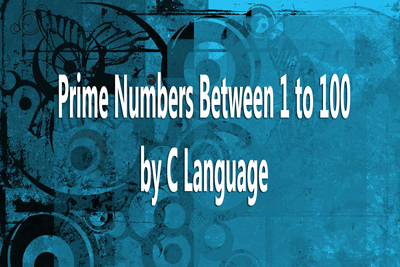Prime no from 1 - 100 - MCA WBUT MAKAUT
12-05-2020    83 times### Write a C program to print the prime numbers between 1 to 100.

If a number has only two factors, 1 & itself, then that number known as Prime Number. In others words, we can say that, a prime no cannot be divided by any numbers between 2 and [that number -1]. As a number cannot be divided by the any number which greater than its half. That is if a number not divide by the number between 2 and half of its numbers, then that number known as prime numbers.

As we know matematically 1 is not a prime number. we create a loop from 2 to 100. Now check each number for prime number. if it is a prime no then print it.

```void main()
{
int N=100,i,d,j,fl;
clrscr();
for(i=2;i<=N;i++)
{
fl=1;
for(d=2;d<=i/2;d++)
{
if(i%d==0)
{
fl=0;
break;
}
}
if(fl)
{
printf("%d  ",i);
}
}
}
```

### Output

2 3 5 7 11 13 17 19 23 29 31 37 41 43 47 53 59 61 67 71 73 79 83 89 97